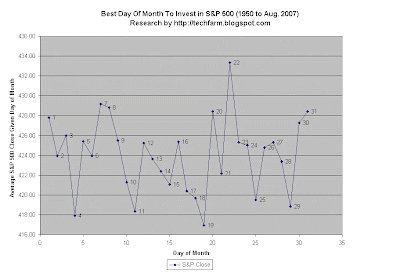## Wednesday, March 19, 2008

### Historical Best Day of Month To Invest or Dollar Cost Average in the S&P 500 from 1950 to 2007

Many people have adopted a Dollar Cost Averaging Strategy of regularly investing in a mutual fund, such as an S&P 500 Index Fund. Often, brokers could give the user an option when they automatically invest the money. Investors could choose which day of the month they can dollar cost average.

Will an investors choice of day to invest on a regular basis affect overall return?In the Chart Above, we have averaged the S&P 500 Closing Average from 1950 to August 2007 given a particular day of month.

We notice that the 19th of the month has the lowest S&P 500 average, suggesting that the 19th of the month during this 1950 to 2007 period is the best day of the month to dollar cost average. The 4th and 11th are the next runners up.

The worst days to invest are the 22nd, followed by the 7th, 8th, 31st, and 20th.

More Data

Day of Month and S&P Average Close:
1. 1 =427.79
2. 2 =423.92
3. 3 =425.97
4. 4 =417.92
5. 5 =425.40
6. 6 =423.93
7. 7 =429.16
8. 8 =428.80
9. 9 =425.51
10. 10 =421.28
11. 11 =418.35
12. 12 =425.22
13. 13 =423.64
14. 14 =422.36
15. 15 =421.07
16. 16 =425.35
17. 17 =420.41
18. 18 =419.70
19. 19 =416.94
20. 20 =428.42
21. 21 =422.16
22. 22 =433.34
23. 23 =425.31
24. 24 =425.00
25. 25 =419.48
26. 26 =424.77
27. 27 =425.30
28. 28 =423.34
29. 29 =418.86
30. 30 =427.28
31. 31 =428.42

Data Ordered by Average S&P 500 Close

Day of Month S&P Close:
1. 19 =416.94
2. 4 =417.92
3. 11 =418.35
4. 29 =418.86
5. 25 =419.48
6. 18 =419.70
7. 17 =420.41
8. 15 =421.07
9. 10 =421.28
10. 21 =422.16
11. 14 =422.36
12. 28 =423.34
13. 13 =423.64
14. 2 =423.92
15. 6 =423.93
16. 26 =424.77
17. 24 =425.00
18. 12 =425.22
19. 27 =425.30
20. 23 =425.31
21. 16 =425.35
22. 5 =425.40
23. 9 =425.51
24. 3 =425.97
25. 30 =427.28
26. 1 =427.79
27. 20 =428.42
28. 31 =428.42
29. 8 =428.80
30. 7 =429.16
31. 22 =433.34

Previous Look at Return by Day of the Month

Kiplinger Article on best Day of Month to Invest

The Average Method

The Averaging Method above is only an approximation. We do realize that S&P 500 index values that have a high value overshadows the days when the S&P 500 had much lower values.Anonymous said...

When you mean x days of the month, are you referring to the calender number or by the business days?

techfarmer said...

Brian: I meant day of the calendar month, such as January 15 (day 15) or November 25 (day 25)Anonymous said...

What if the 19th falls on a Sat. would it be a good idea to average cost on the 18th Friday or the 21st Monday?

thanks

techfarmer said...

@Brian: I think most mutual funds and ETFs allow you to invest Mon-Friday only.

I'll have to revisit this research in the future. For one thing, the research above overweights the times when the S&P 500 had a high value (such as 1500) and underweights the times when the S&P 500 had a lower value.

John said...

Did you ever conclude research from Brian's question: If the optimum day to invest falls on a weekend. Is there a next best day of the week (either Friday or Monday) that is proven to be a great day to invest?

John said...

Did you ever conclude research from Brian's question: If the optimum day to invest falls on a weekend. Is there a next best day of the week (either Friday or Monday) that is proven to be a great day to invest?

techfarmer said...

When I do have time, I would like to do more research with (hopefully) improved methodology.

techfarmer said...

I finally updated the Best Day of Month to Invest or Dollar Cost Average from 1950 to 2014.

It uses a better methodology that doesn't overweight the S&P 500 when the S&P 500 was high.

Here it is:

http://techfarm.blogspot.com/2014/07/best-day-of-month-to-dollar-cost.html

 Enter your search terms Submit search form